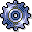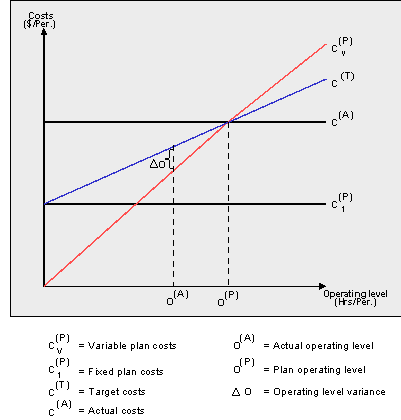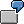Activity-Dependent Primary Cost PlanningUse

You plan activity-dependent primary costs as follows on a cost center(s)/cost center group:

• By cost element(s)/cost element group
• Based on the activity type(s)/activity type group

This means that you can plan a fixed amount and a variable amount for your primary costs. Variable costs are the costs incurred in producing a given activity, proportional to the quantity of activity produced. You can calculate target costs based on the costs split and the operating rate (actual activity quantity related to the plan activity quantity). Target costs for costs planned by value are comprised of:

• Plan fixed costs
• Plan variable costs adapted to the actual operating level (= target costs of the actual operating level)You plan salaries on a production cost center based on the manufacture of the finished product X. The planned activity type is Production hours FST. Your activity-dependent primary cost planning, for example, using planner profile SAP101 and standard planning layout 1 - 101) contains the following values:

Activity Type FST: 1000 hrs

 Cost element Total costs Fixed costs 430000 \$600,000 \$200,000

To produce 1000 hours of activity type FST, costs of \$400,000 are required. This amount represents the variable costs. The portion of fixed costs, \$200,000, is incurred irrespective of the activity quantity.

For activity-dependent costs, which are planned based on quantity, the following formulas apply:

Variable target quantity = Variable plan quantity X Operating rate

Fixed target costs = Fixed target quantity X Fixed price

+ Variable target quantity X Fixed price

+ Fixed target quantity X Variable price

Variable target costs = Variable target quantity X Variable price